# 具有Holling Ⅳ型功能反应捕食系统的状态反馈控制*

(陕西科技大学 文理学院， 西安 710021)

## 1 模型的建立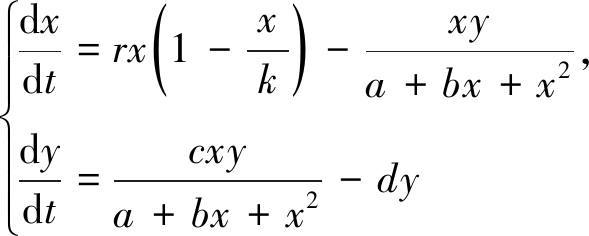(1)

(b) 如果d1=0,0<d3<k，系统(1)有三个平衡点：鞍点(0,0)、稳定结点(k,0)和唯一正平衡点(x0,y0)，而且系统(1)没有闭轨．当kd4时，(x0,y0)是鞍结点；当k=d4时，(x0,y0)是尖点．其中，x0=d3y0=r(1-x0/k)(a+bx0+x02)．

(c) 如果d1>0,d2>0，系统(1)最多有四个平衡点：(0,0)，(k,0)，(x1,y1)和(x2,y2)．当kx1时，系统(1)只有两个平衡点：鞍点(0,0)，稳定结点(k,0)；当x1<kx2时，有三个平衡点：鞍点(0,0)和(k,0)，焦点或结点(x1,y1)；当k>x2时，有四个平衡点：鞍点(0,0)和(x2,y2)，稳定结点(k,0)和k<d0(k>d0)的稳定(不稳定)焦点或结点(x1,y1)． 其中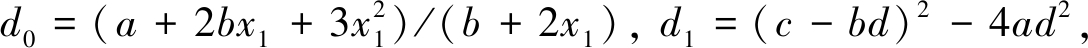d2=c-bd, d3=(c-bd)/2d, d4=2d3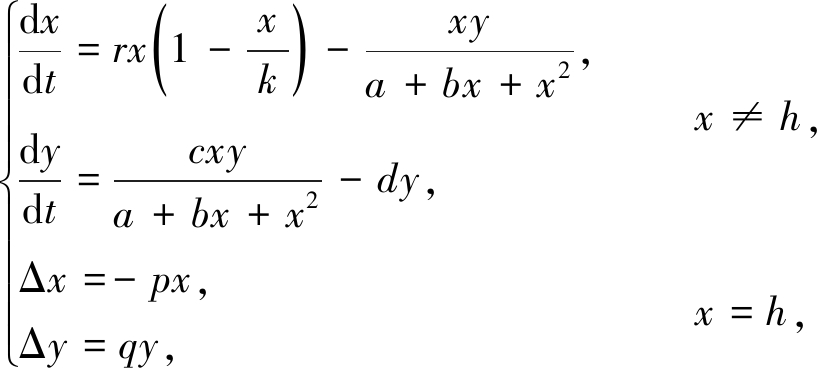(2)

## 2 定 性 分 析

### 2.1 半平凡周期解的存在性和稳定性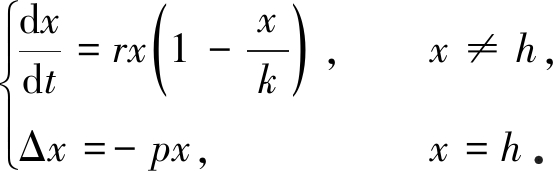x0=x(0)=(1-p)h，logistic方程x′=rx(1-x/k)的解为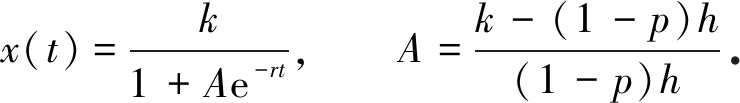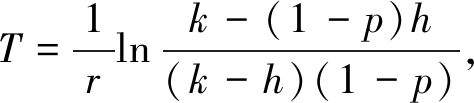x(T)=hx(T+)=x0．于是，系统(2)存在半平凡周期解：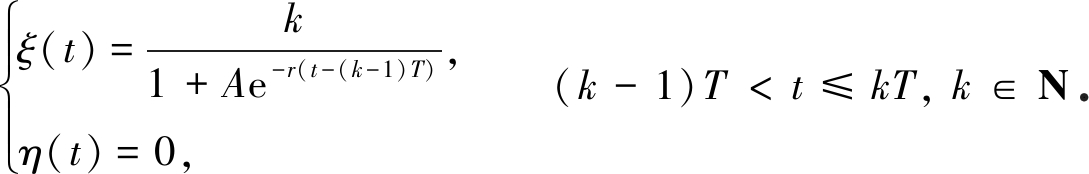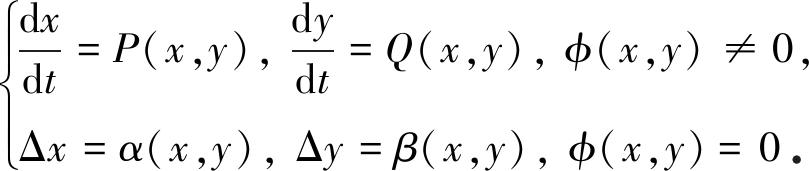(3)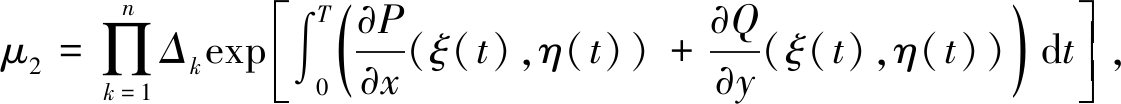(4)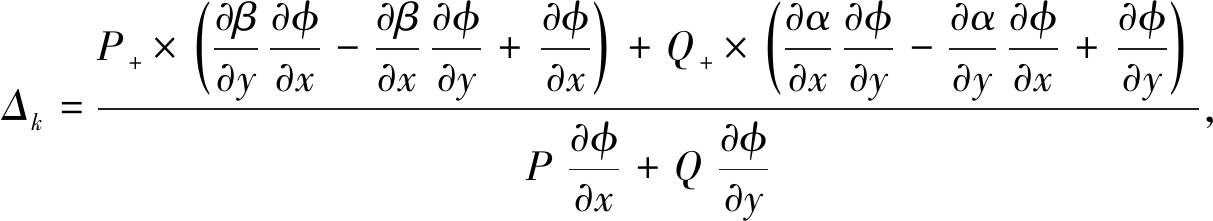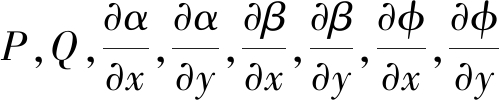在点(ξ(τk),η(τk))处取值，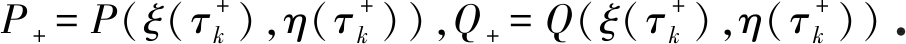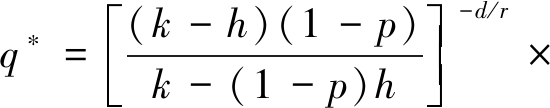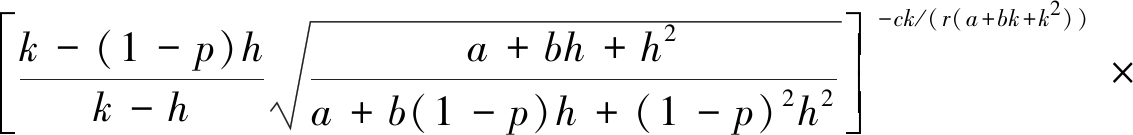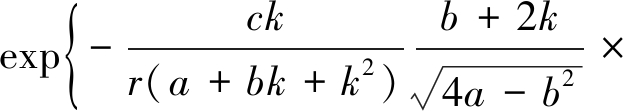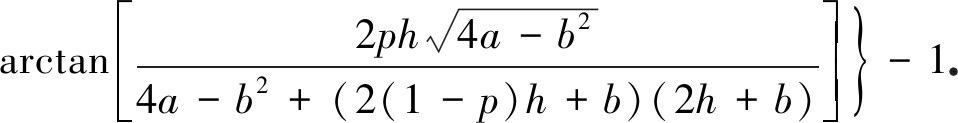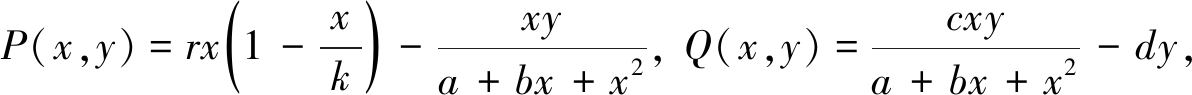α(x,y)=-px, β(x,y)=qy, φ(x,y)=x-h,

(ξ(T),η(T))=(h,0), (ξ(T+),η(T+))=((1-p)h,0)．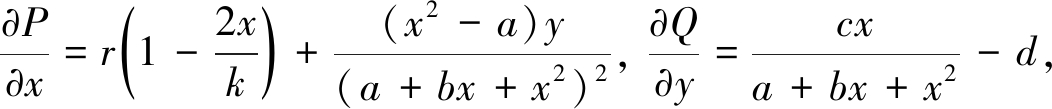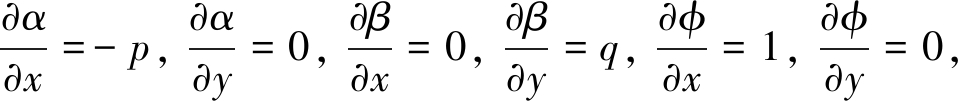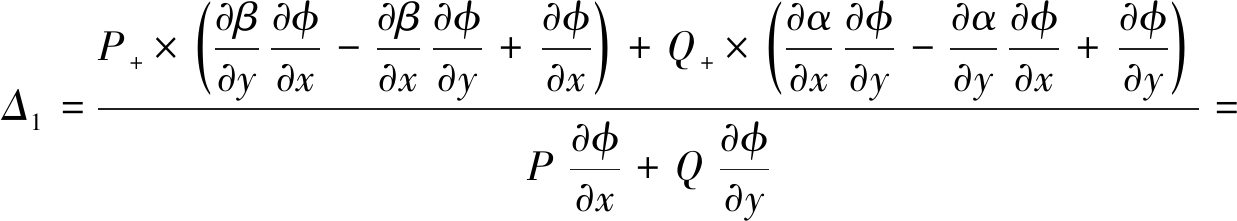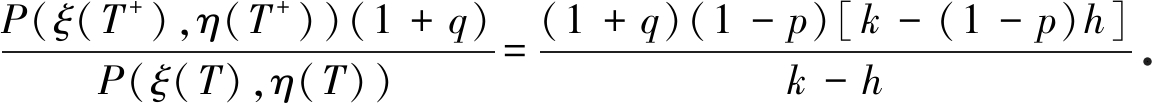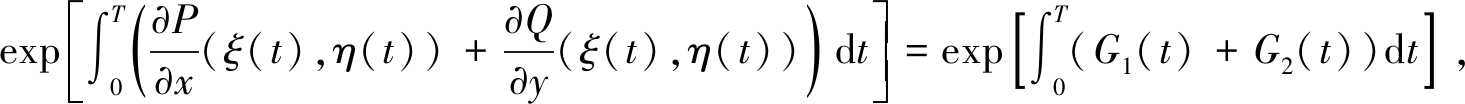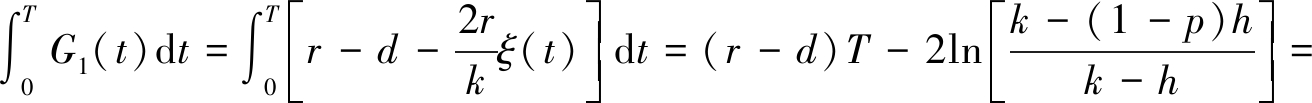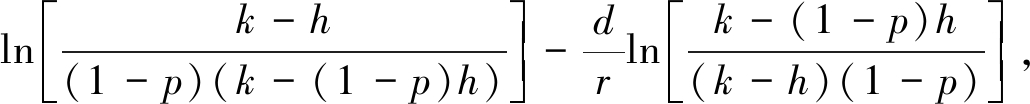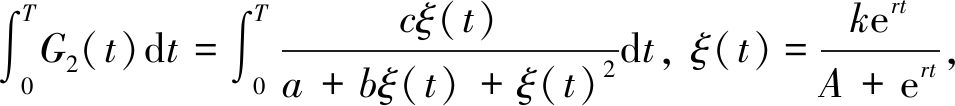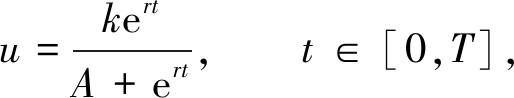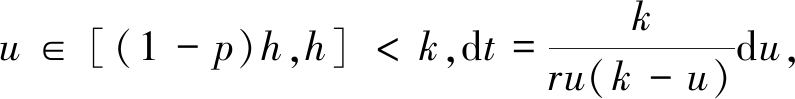并且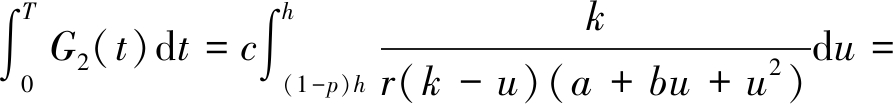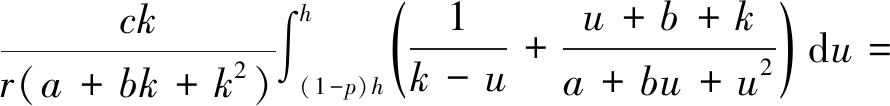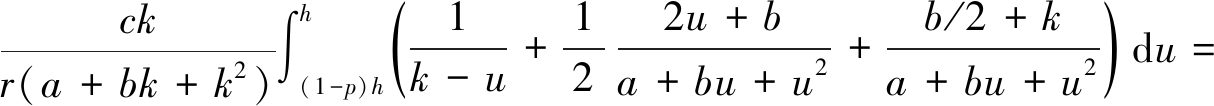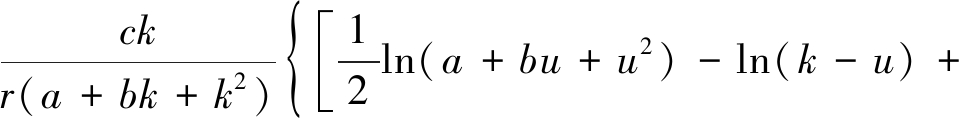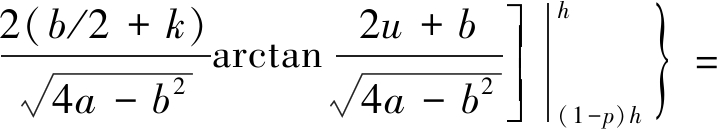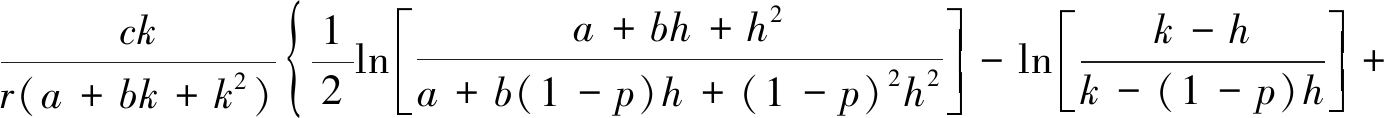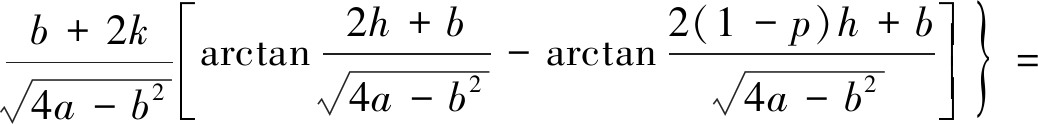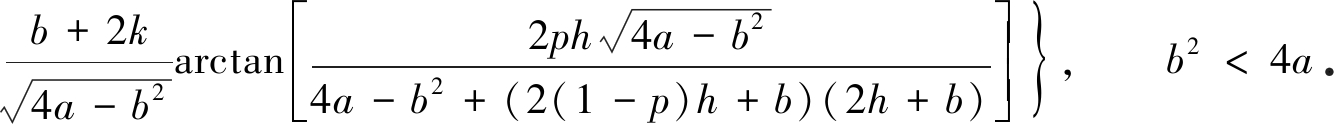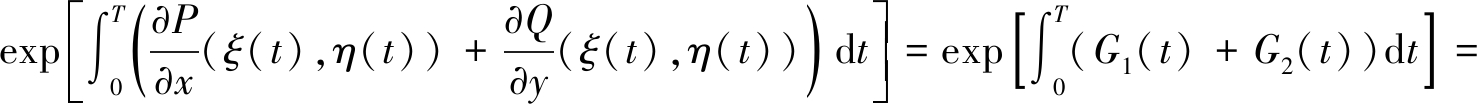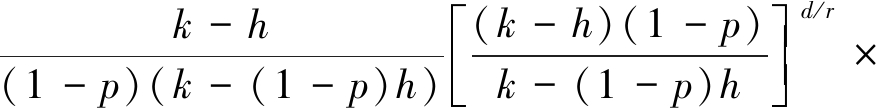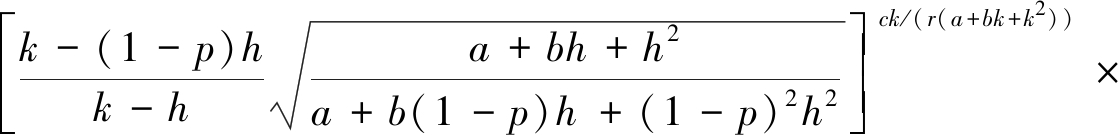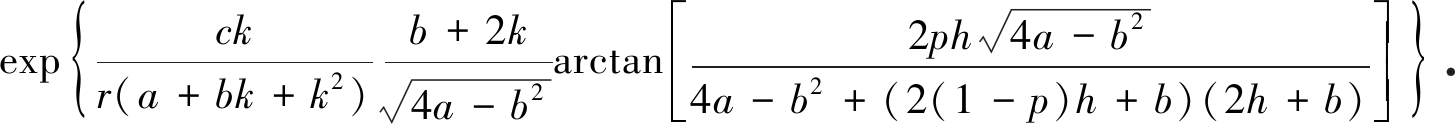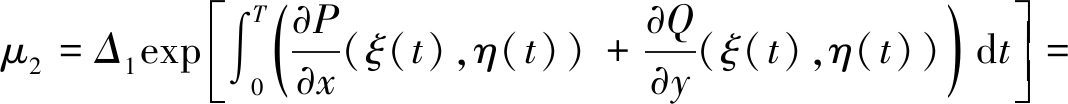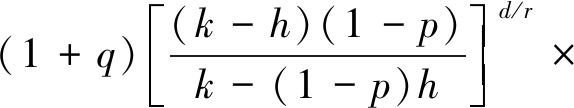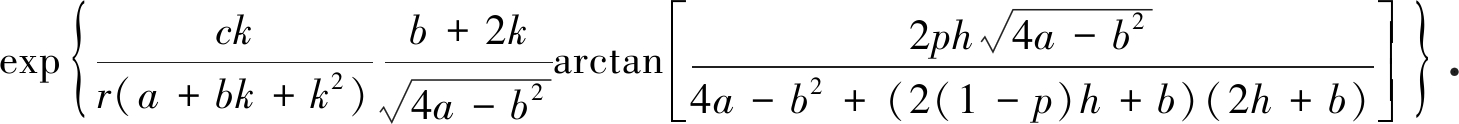q=q*时，系统(2)出现fold分岔．

δy1=f(qy0)=(1+q)v(Ty0=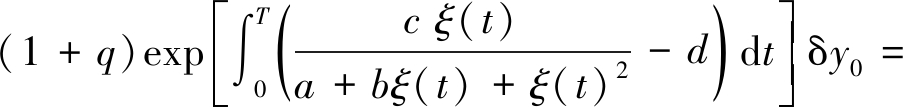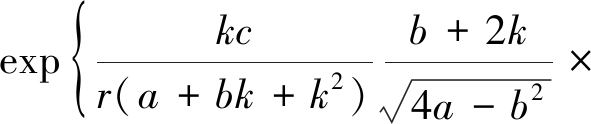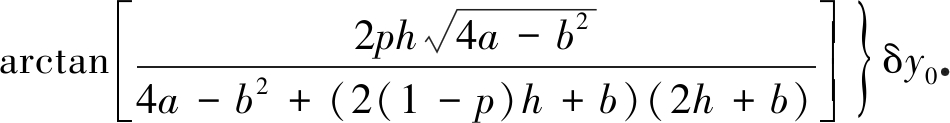### 2.2 阶1-周期解的存在性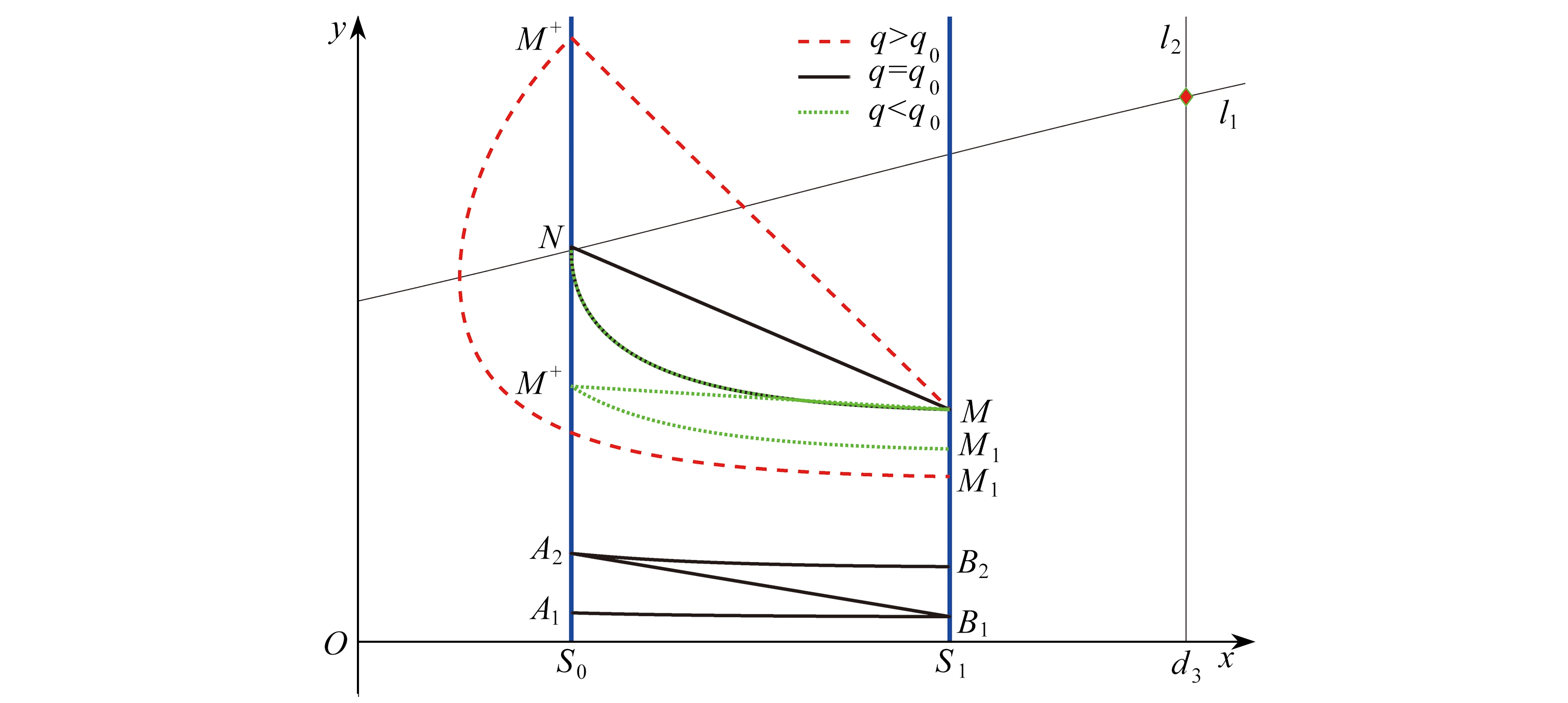Fig. 1 The existence of the order-1 periodic solution to system (2) for h<d3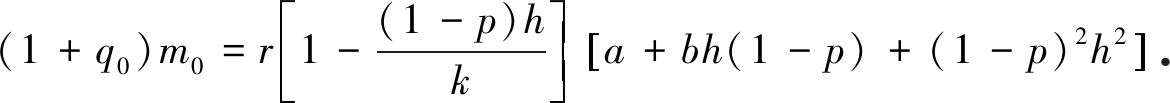1) 如果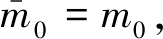那么轨道NMN即为系统(2)的阶1-周期解．

2) 如果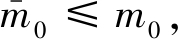M的后继函数满足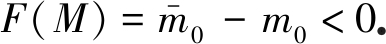由后继函数的连续性知，在脉冲集S1上点M和点B1之间至少存在一点C， 使得F(C)=0，即系统(2)存在阶1-周期解． 证毕．

## 3 数 值 模 拟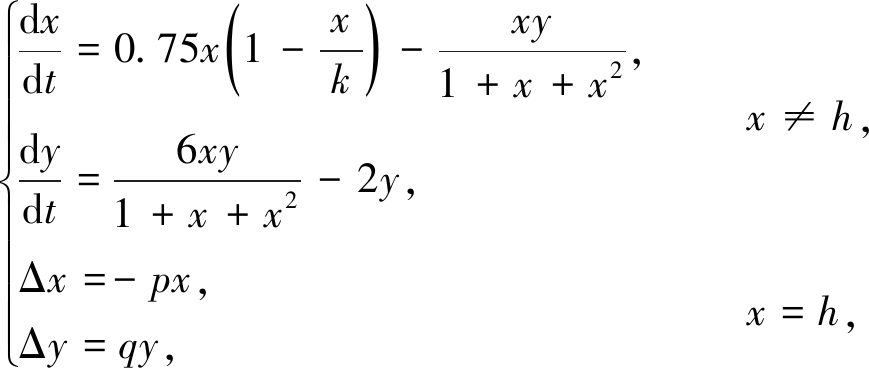(5)

p=0.6，h=0.8时，系统(5)的两个Poincaré截面分别为S0={(x,y)|x=0.8,y≥0}，S1={(x,y)|x=0.32,y≥0}．当k分别取1.25，2和3时，由定理1可得q*分别为0.776 6，0.600 1和0.534 6．如图3(a)～(c)所示，当q*>q=0.3且k=1.25,2和3时，系统(5)过初值(0.3,0.1)的解随着时间的增加而趋于半平凡周期解，即半平凡周期解是稳定的；当q*<q=1时，半平凡周期解失去稳定性，通过fold分岔，分岔出阶1-周期解(见图3(d)～(f))．显然，数值模拟有效地验证了定理1的正确性．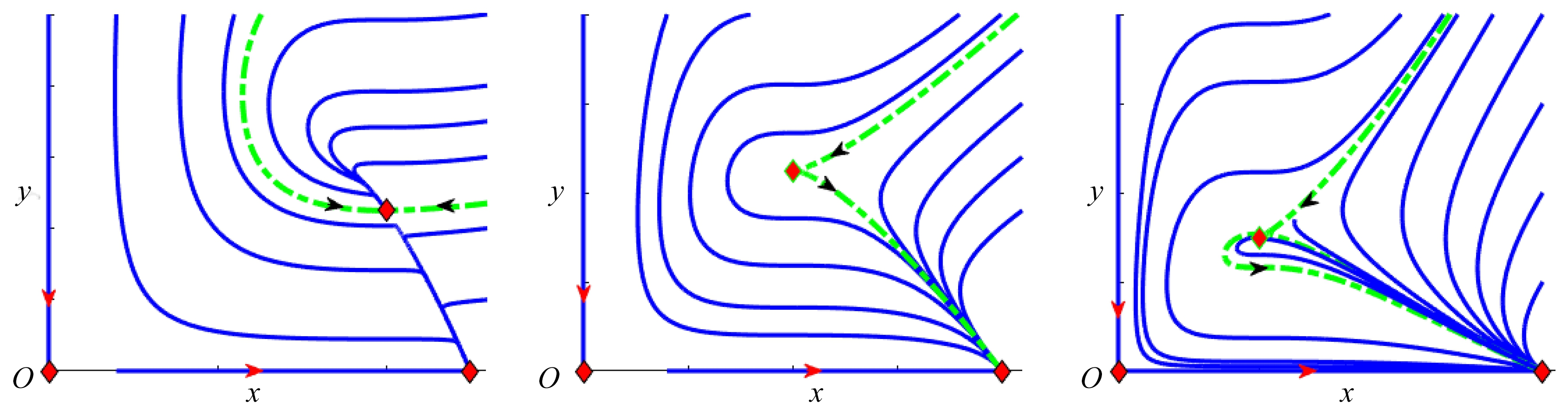(a) k=1.25 (b) k=2 (c) k=3

Fig. 2 The phase portraits of system (5) without state feedback control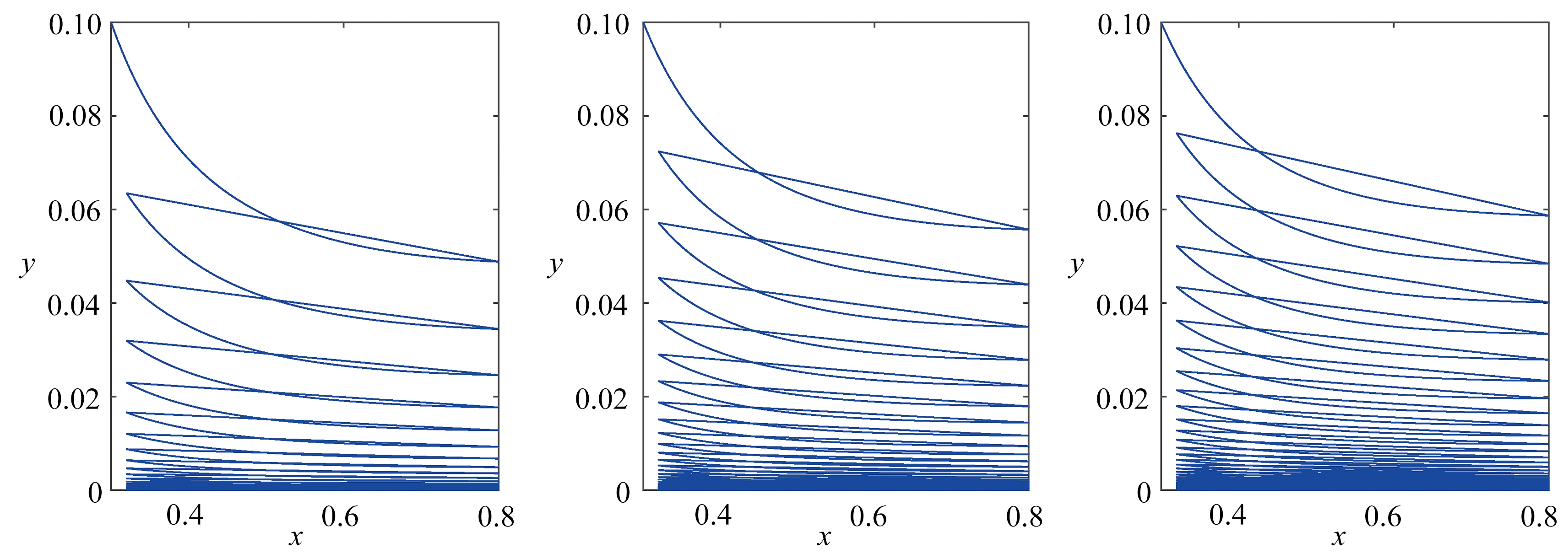(a) k=1.25, q=0.3 (b) k=2, q=0.3 (c) k=3, q=0.3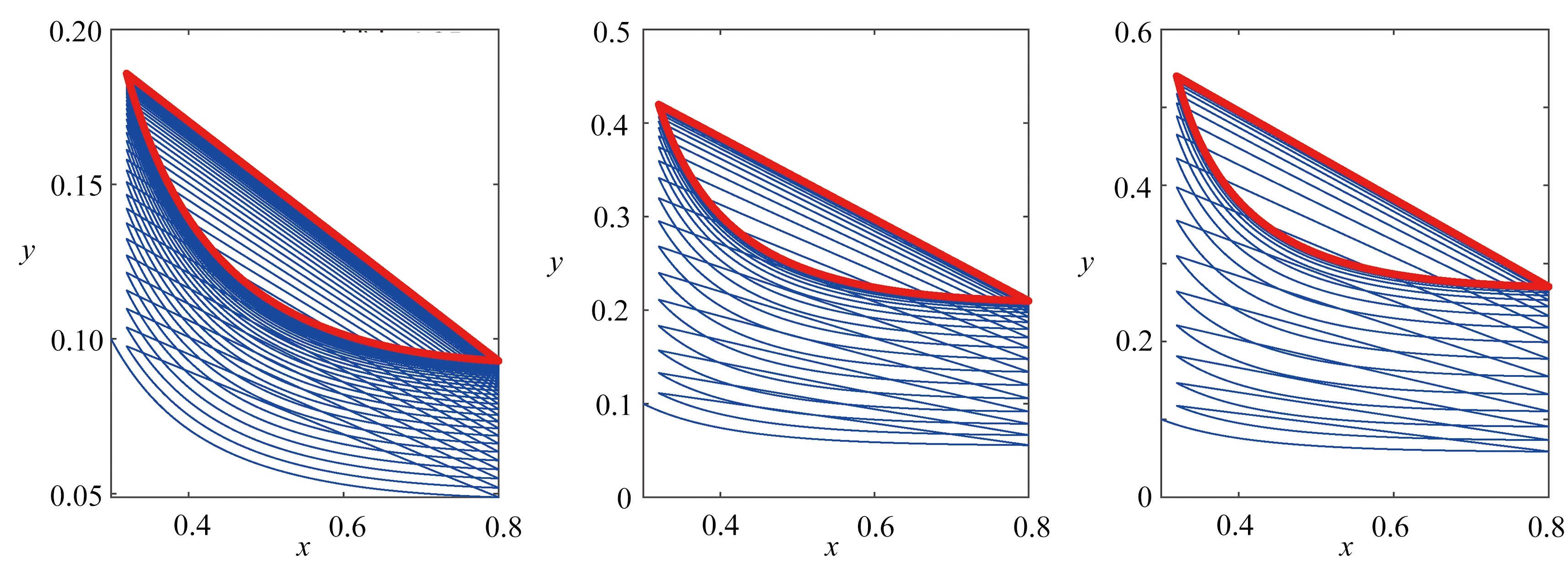(d) k=1.25, q=1 (e) k=2, q=1 (f) k=3, q=1

Fig. 3 The phase portraits of system (5) with q=0.3 and q=1 for k=1.25,2,3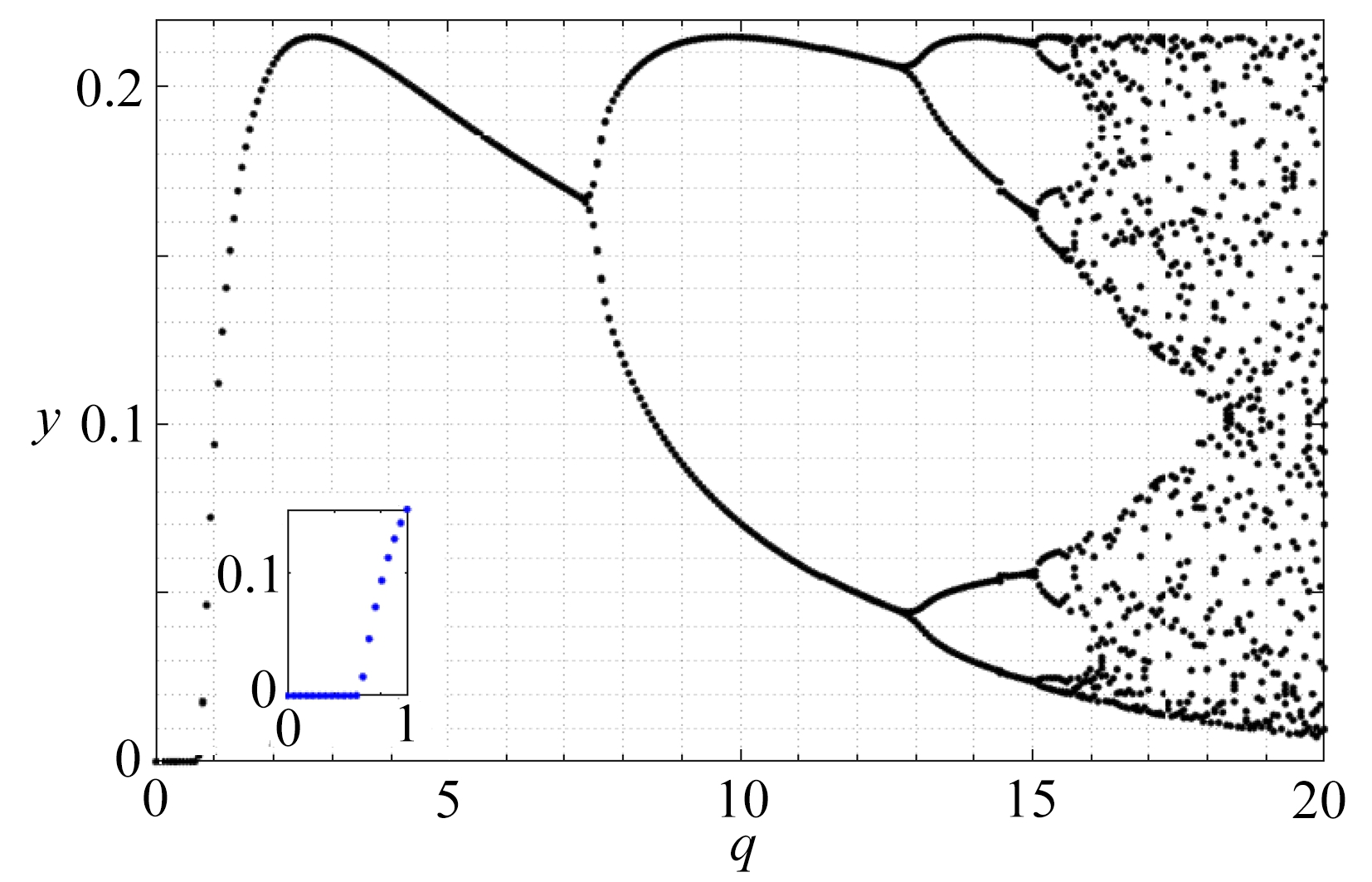(a) k=1.25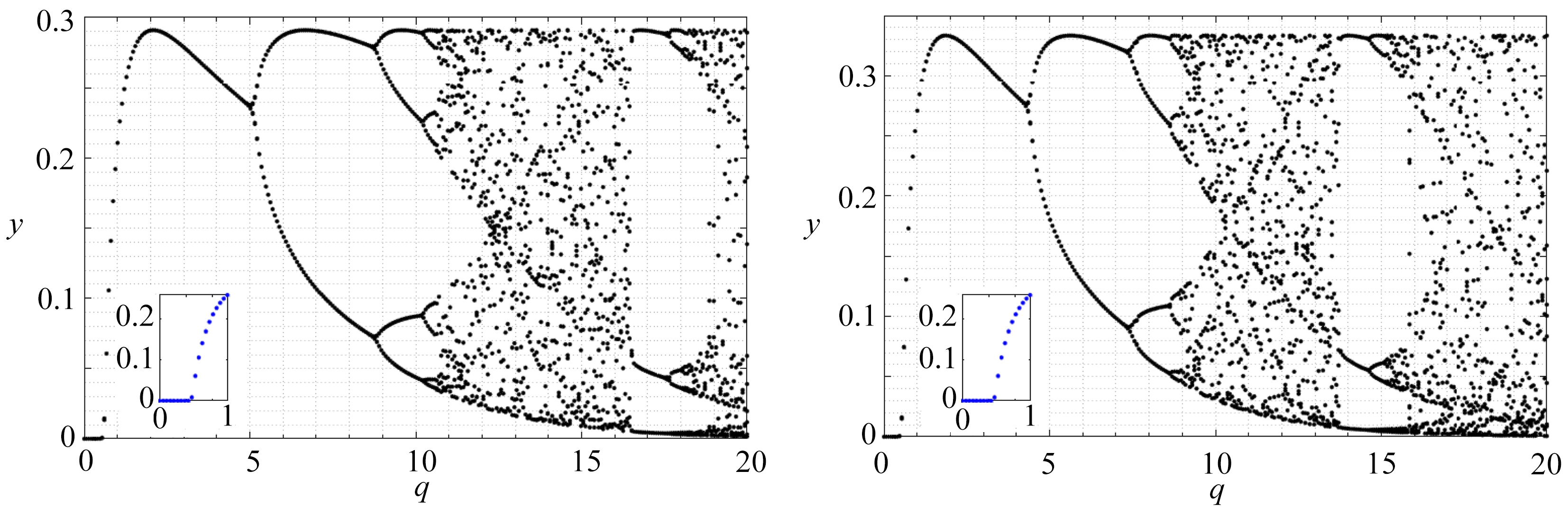(b) k=2 (c) k=3

Fig. 4 The bifurcations of system (5) for k=1.25,2,3 and q∈[0,20]

Table 1 Critical bifurcation values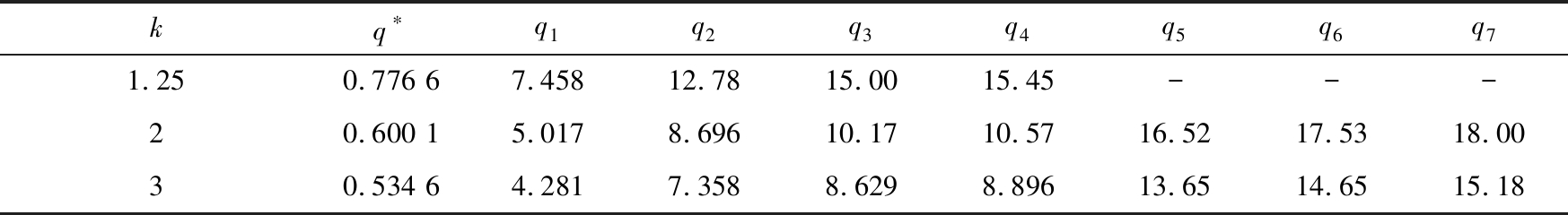kq∗q1q2q3q4q5q6q71.250.776 67.45812.7815.0015.45---20.600 15.0178.69610.1710.5716.5217.5318.0030.534 64.2817.3588.6298.89613.6514.6515.18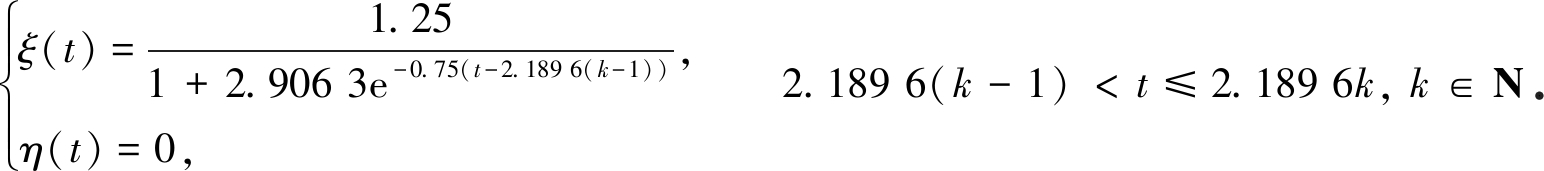q足够小时，半平凡周期解(ξ(t),η(t))是稳定的，该周期解保持其稳定性直到达到临界点q*．在qq*处，半平凡周期解通过fold分岔分岔出阶1-周期解．该阶1-周期解在q∈(q*,q1)时是稳定的，而在q>q1时是不稳定的．即当q增加到q1时，阶1-周期解失稳，出现阶2-周期解；当q增大到q2时，出现阶4-周期解，周期翻倍；当q达到q3时，出现阶8-周期解，周期再翻倍，这种现象叫做倍周期分岔(flip分岔)；当q达到q4时，倍周期分岔现象突然中断，过渡到混沌运动状态．

## 4 总 结

 马知恩. 种群生态学的数学建模与研究[M]. 合肥: 安徽教育出版社, 1996.(MA Zhien. Mathematical Modeling and Research of Population Ecology[M]. Hefei: Anhui Education Press, 1996.(in Chinese))

 LIU X X, HUANG Q D. The dynamics of a harvested predator-prey system with Holling type Ⅳ functional response[J]. Biosystems, 2018, 169/170: 26-39.

 LAJMIRI Z, KHOSHSIAR G R, ORAK I. Bifurcation and stability analysis of a ratio-dependent predator-prey model with predator harvesting rate[J]. Chaos, Solitons & Fractals, 2018, 106: 193-200.

 SEN M, SRINIVASU P D N, BANERJEE M. Global dynamics of an additional food provided predator-prey system with constant harvest in predators[J]. Applied Mathematics and Computation, 2015, 250: 193-211.

 LI S Y, XIONG Z L, WANG X. The study of a predator-prey system with group defense and impulsive control strategy[J]. Applied Mathematical Modelling, 2010, 34(9): 2546-2561.

 TIAN Y, TANG S Y, CHEKEC R A. Dynamic complexity of a predator-prey model for IPM with nonlinear impulsive control incorporating a regulatory factor for predator releases[J]. Mathematical Modelling and Analysis, 2019, 24(1): 134-154.

 焦建军, 陈兰荪, J·J·尼托, 等. 连续收获捕食者与脉冲存放食饵的阶段结构捕食-食饵模型的全局吸引和一致持久[J]. 应用数学和力学, 2008, 29(5): 589-600.(JIAO Jianjun, CHEN Lansun, NIETO J J, et al. Permanence and global attractivity of a stage-structured predator-prey model with continuous harvesting on predator and impulsive stocking on prey[J]. Applied Mathematics and Mechanics, 2008, 29(5): 589-600.(in Chinese))

 蒋贵荣, 刘期怀, 龙腾飞, 等. 脉冲动力系统的分岔混沌理论及其应用[M]. 北京: 科学出版社, 2015.(JIANG Guirong, LIU Qihuai, LONG Tengfei, et al. Bifurcation Chaos Theory of Impulsive Dynamic Systems and Application[M]. Beijing: Science Press, 2015.(in Chinese))

 YANG J, TANG G Y, TANG S Y. Holling-Tanner predator-prey model with state-dependent feedback control[J]. Discrete Dynamics in Nature and Society, 2018.

DOI: 10.1155/2018/3467405.

 HE Z M. Impulsive state feedback control of a predator-prey system with group defense[J]. Nonlinear Dynamics, 2015, 79(4): 2699-2714.

 钱临宁, 陆启韶. 一类自治脉冲微分方程的动力学研究[J]. 动力学与控制学报, 2008, 6(2): 97-101.(QIAN Linning, LU Qishao. Dynamics of a class of autonomous impulsive equations[J]. Journal of Dynamics and Control, 2008, 6(2): 97-101.(in Chinese))

 白露, 刘琼, 陈武大仁. 一类捕食-食饵系统的状态依赖反馈控制模型[J]. 应用数学进展, 2018, 7(10): 1340-1348.(BAI Lu, LIU Qiong, CHEN Wudaren. A predator-prey model with state-dependent feedback control[J]. Advances in Applied Mathematics, 2018, 7(10): 1340-1348.(in Chinese))

 LIANG Z Q, ZENG X P, PANG G P, et al. Periodic solution of a Leslie predator-prey system with ratio-dependent and state impulsive feedback control[J]. Nonlinear Dynamics, 2017, 89(4): 2941-2955.

 ZHOU Z W, LIANG Z Q, ZENG X P, et al. Periodic solution of Holling-Tanner model with impulsive state feedback control[J]. Mathematica Applicata, 2017, 30(3): 576-588.

 HUANG J C, XIAO D M. Analyses of bifurcations and stability in a predator-prey system with Holling type-Ⅳ functional response[J]. Acta Mathematicae Applicatae Sinica, 2004, 20(1): 167-178.

 陈兰荪. 害虫治理与半连续动力系统几何理论[J]. 北华大学学报(自然科学版), 2011, 12(1): 1-9.(CHEN Lansun. Pest control and geometric theory of semi-continuous dynamical system[J]. Journal of Beihua University (Natural Science), 2011, 12(1): 1-9.(in Chinese))

 张建树. 前沿与交叉科学-混沌生物学[M]. 西安: 陕西科学技术出版社, 1998.(ZHANG Jianshu. Frontiers and Crossover Science-Chaos Biology[M]. Xi’an: Shaanxi Science and Technology Press, 1998.(in Chinese))

# State Feedback Control of Predator-Prey Systems With Holling Ⅳ Functional Responses

WANG Xiaoe, LIN Xiaolin, LI Jianquan

(School of Arts and Sciences, Shaanxi University of Science and Technology, Xian 710021, P.R.China)

Abstract: A class of predator-prey systems with Holling Ⅳ functional responses under state feedback control were studied. The sufficient conditions for the existence and stability of semi-trivial solutions and order-1 periodic solutions were obtained by means of the analogue of the Poincaré criterion and the geometric theory for semi-continuous dynamical systems. The numerical simulation verifies the correctness of the conclusion and the effectiveness of the state feedback control, and reveals abundant dynamic behaviors of the state feedback control system, such as the fold bifurcation, the flip bifurcation and chaos.

Key words: Holling Ⅳ functional response; state feedback control; successor function; order-1 periodic solution

DOI: 10.21656/1000-0887.400314

* 收稿日期： 2019-10-05； 修订日期：2020-05-16

Foundation item: The National Natural Science Foundation of China(11971281)